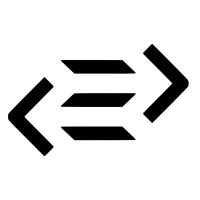1×
Become a member
to unlock all featuresLevel Up!

Access all courses & lessons on egghead today and lock-in your price for life.

Autoplay

Foldl in PureScriptPureScript
0.11.7 - ^0.12

Folds in PureScript take a List, Array, or some datatype and recursively apply a function to each item which will produce us a single value.

In this lesson, we are going to see what folds are and how they are used to fold over arrays and other types by defining our own fold function.CodeYou must be a Member to view code

Access all courses and lessons, track your progress, gain confidence and expertise.

Become a Member
and unlock code for this lessonDiscussTranscript

Instructor: We'll explain foldL by creating our own function. We'll call that foldL tick, and its type definition is forall a b, with a function of b -> a -> b. That goes to b, to a list of a, and returns us a b. On the next line, we'll do foldL tick f, arc, and L, and then that equals. Now we'll case over L. Say case L of.

Then some pattern matching. If our L is nil, we'll return arc. If not, we'll do X cons Xs. We'll recursively call foldL. We'll pass in F, then the results of calling F, arc, and X. At the end, we'll pass in Xs. Let's put that in use.

We'll go down here. We'll do fold tick, and we'll pass in a function of plus. Plus is a type of b -> a -> b, which is what we need for our fold. We'll pass our fold an initial value, which is 0Then, at the end, we'll pass it the list we want to go over, which is myIntList.

Let's go through what happens when you run a function. First, we're casing over L, which is our list. Does our list equal nil? No. Now we'll split that apart with X cons Xs. Our X will be 1, and our Xs will be 2, 3, 4, and nil.

We'll recursively call on foldL tick, and we'll pass it our function, which is plus. Our arc is 0and our X is 1, and that turns into 01, which equals 1. Then we pass in the Xs, which is 2, 3, 4, and nil.

We'll go back to our recursive call of foldL tick. Does L equal nil? No, we just passed in 2, 3, 4, so we'll go on to the next part. Now we'll cons over 2, 3, 4, so X will equal 2, and Xs would be 3, 4, and nil. Now we're doing arc + X, which is 2, which 1 + 2 = 3. We're passing our Xs, which is 3, 4, and nil.

At that point, our arc is 3. Then we cons over 3, 4, so our X would be 3 and Xs would be 4 and nil. We'll fold L, F. Arc is 3 and our X is three, so 3 + 3 = 6. We'll pass in our Xs, which if 4 and nil. Our arc is now 6, and there's still no nil for L. So X cons Xs would actually be 4 for the X, and the Xs would be nil.

This time, we do arc Xs, so our arc is 6, plus X is 4, so that makes 10. Then, the second value we pass in is nil. Again, we case on L, and finally, L is nil, so we need to return arc. By now, arc has accumulated to 10, so we return 10.

It should make a little more sense how foldL is used when we pass in a function, which in our case was plus, which has a type of b -> a -> b. We pass an initial value, which is 0and then we pass our list. As foldL recursively calls, we keep accumulating the numbers. It's like doing 1 + 2 + 3 + 4, but plus can be replaced with anything that has a type b -> a -> b.